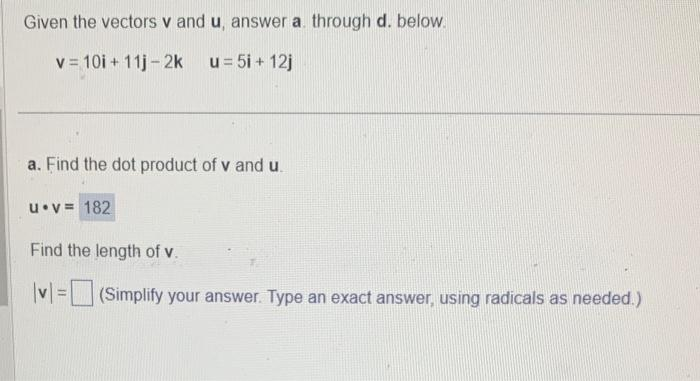# (Solved): Given the vectors $$\mathbf{v}$$ and $$\mathbf{u}$$, answer a. through $$\boldsymbol{d}$$. be ...Given the vectors $$\mathbf{v}$$ and $$\mathbf{u}$$, answer a. through $$\boldsymbol{d}$$. below. $\mathbf{v}=10 \mathbf{i}+11 \mathbf{j}-2 \mathbf{k} \quad \mathbf{u}=5 \mathbf{i}+12 \mathbf{j}$ a. Find the dot product of $$v$$ and $$u$$. $u \cdot v=$ Find the length of $$v$$. $$|v|=\quad$$ (Simplify your answer. Type an exact answer, using radicals as needed.)

We have an Answer from Expert